# RD Sharma Solutions For Class 12 Maths Exercise 19.16 Chapter 19 Indefinite Integrals

RD Sharma Solutions for Class 12 Maths Exercise 19.16 Chapter 19 Indefinite Integrals is provided here. Students who are willing to clear their doubts and improve their problem-solving skills can make use of RD Sharma Solutions for Class 12. This is a very useful resource for students to prepare confidently for their examinations.

All these RD Sharma Solutions are prepared by subject experts at BYJU’S, on par with the latest examination patterns. This exercise deals with integrals reducible to the form

$$\begin{array}{l}\int \frac{1}{ax^{2} + bx + c} \ dx\end{array}$$
with algorithm. To know more about this topic, students can refer to RD Sharma Solutions for Class 12 Maths Chapter 19 Exercise 19.16.

## Download the PDF of RD Sharma Solutions For Class 12 Chapter 19 – Indefinite Integrals Exercise 19.16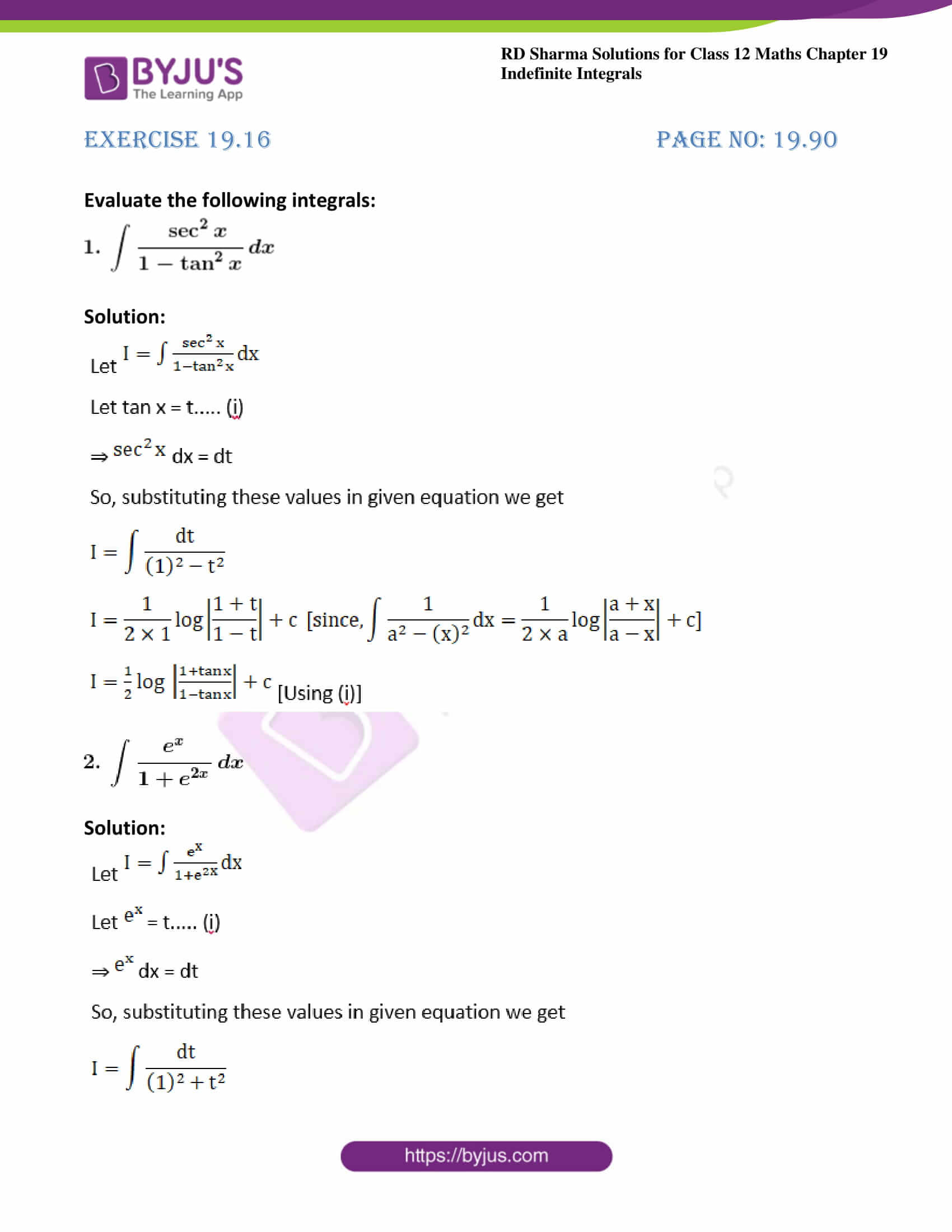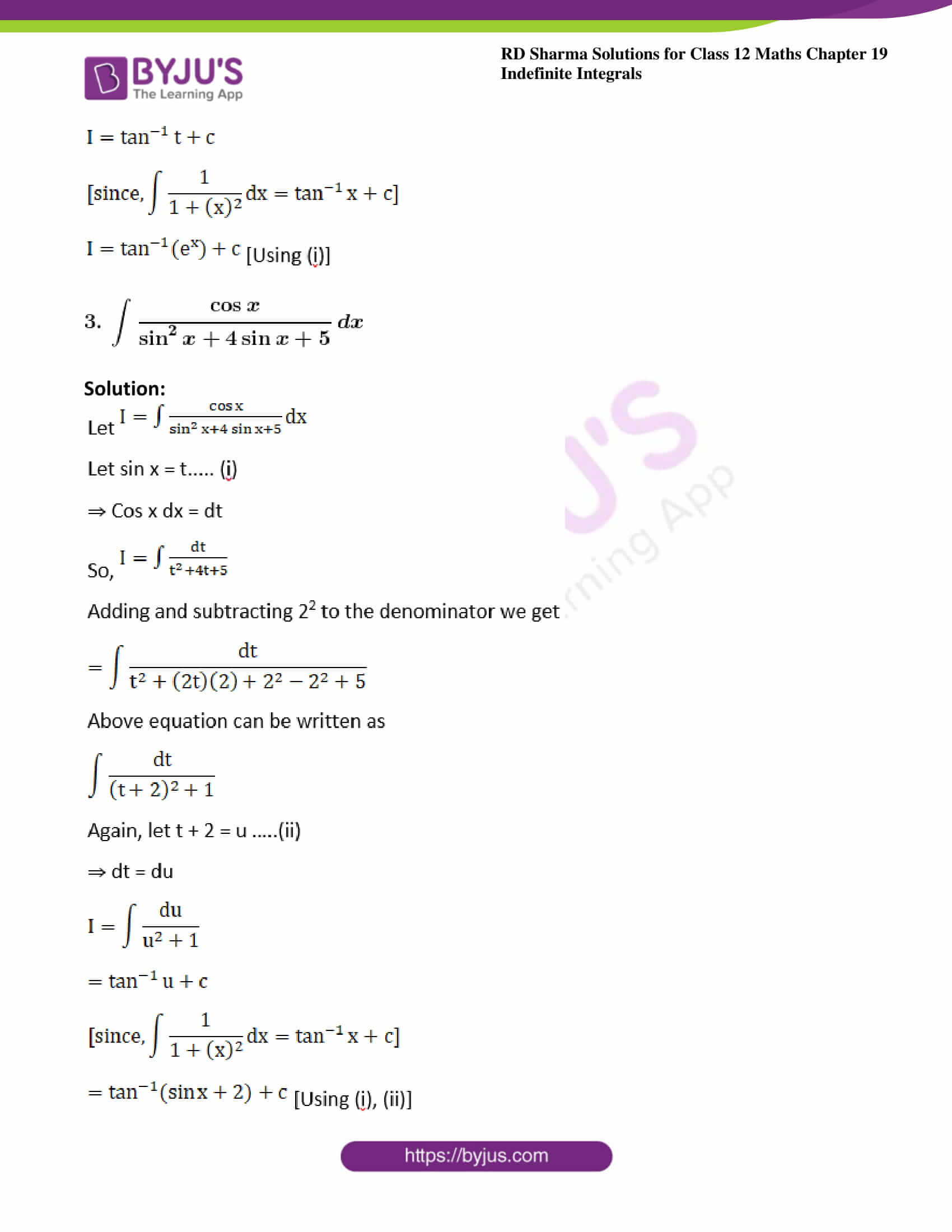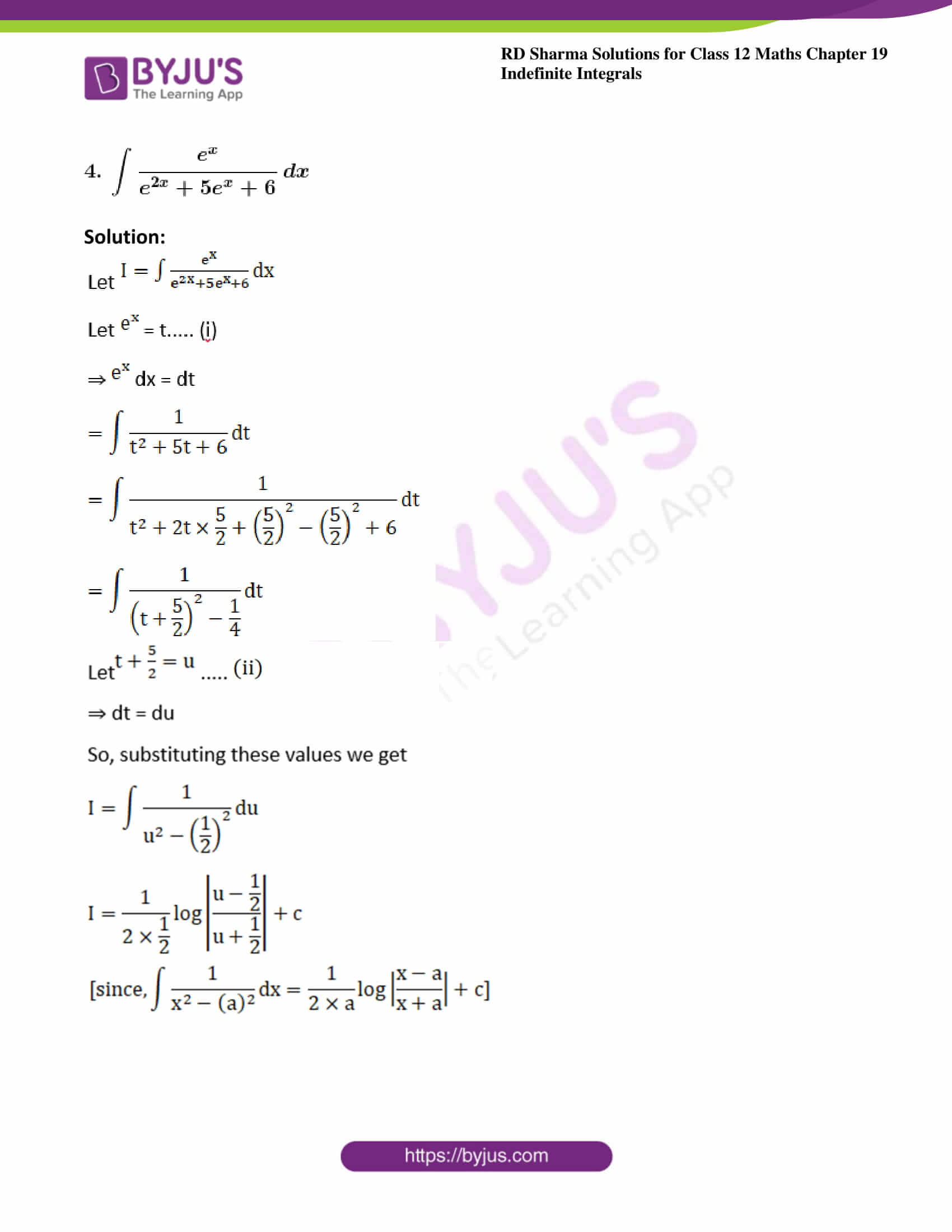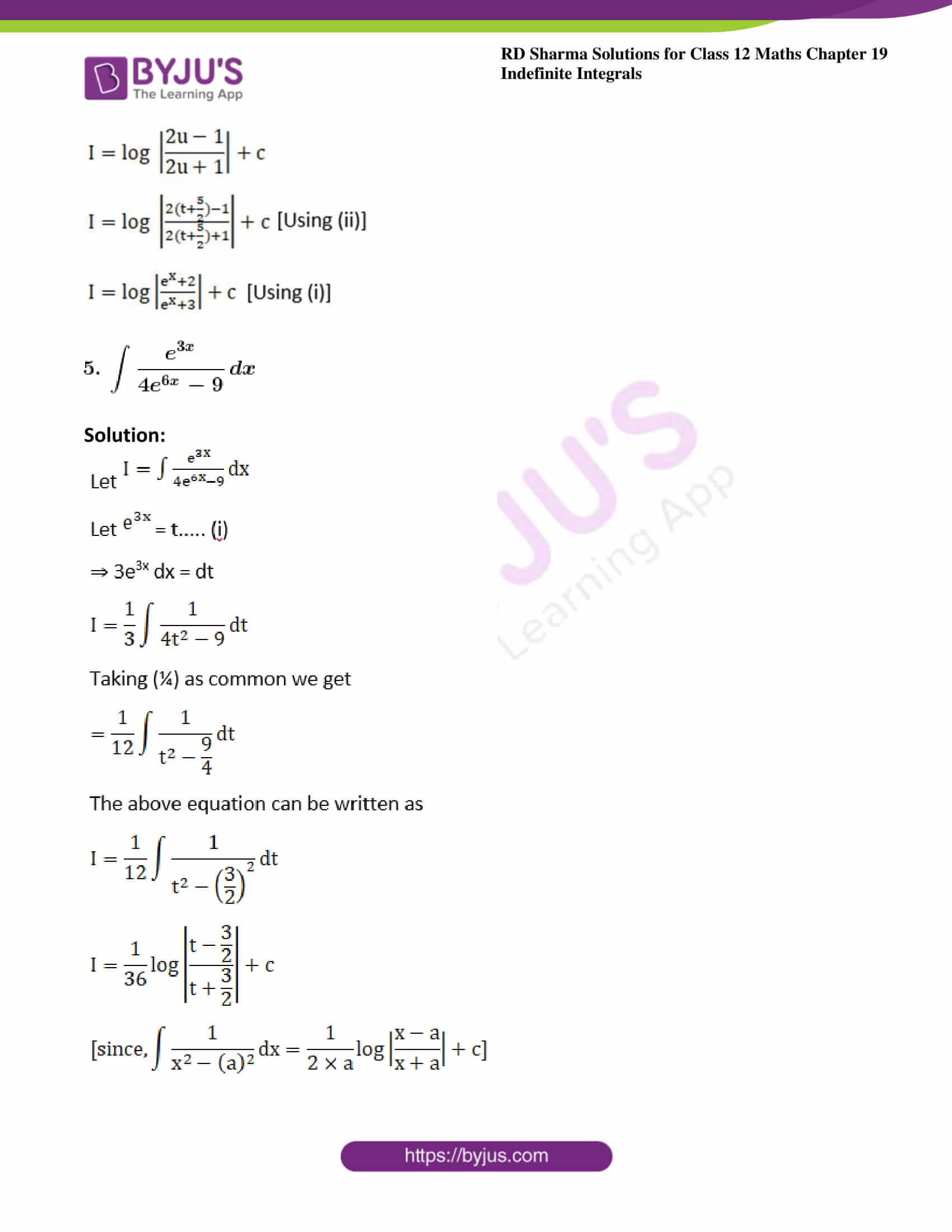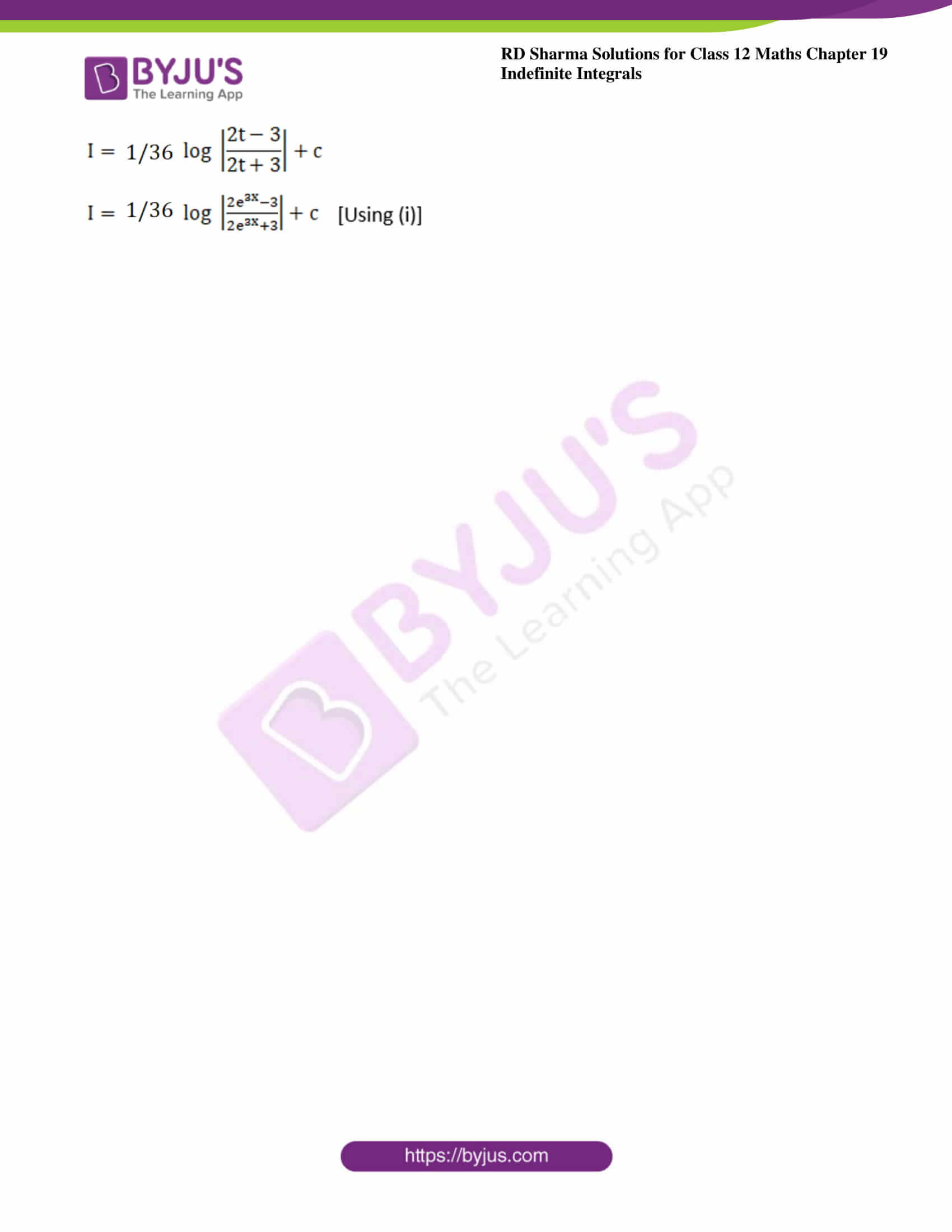### Exercise 19.16 Page No: 19.90

Evaluate the following integrals: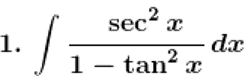Solution: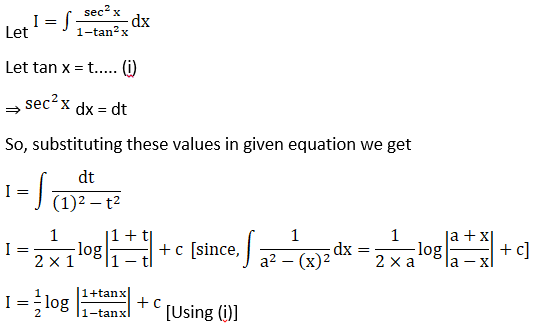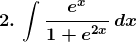Solution: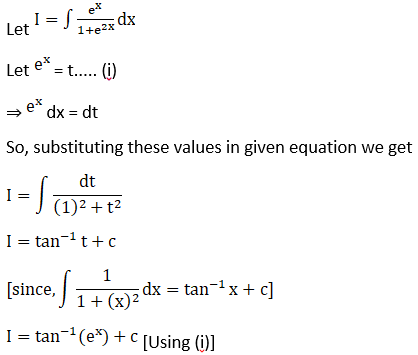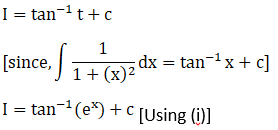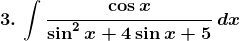Solution: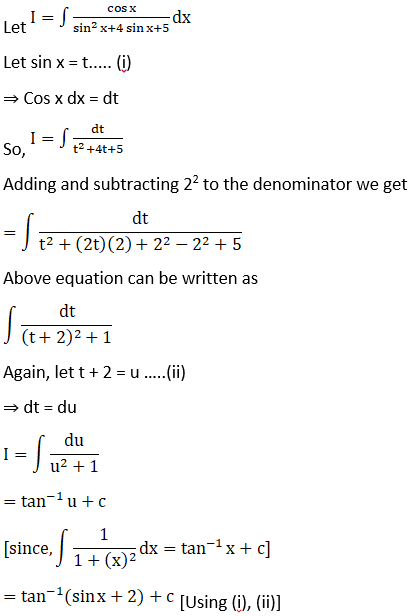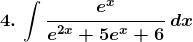Solution: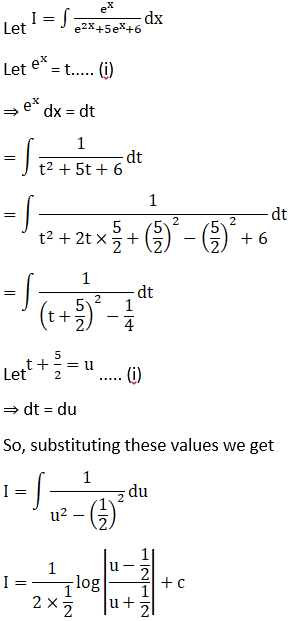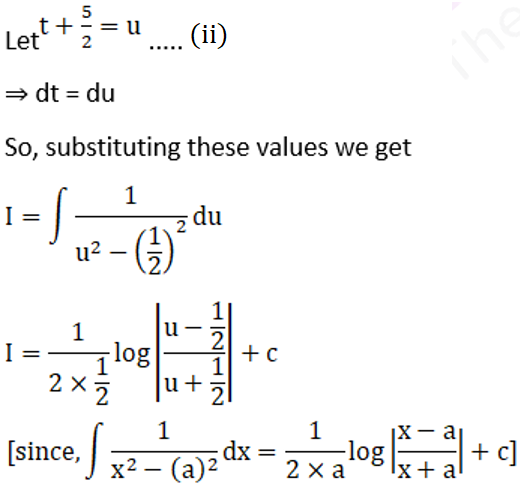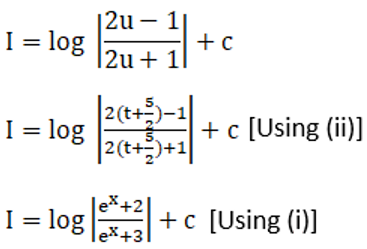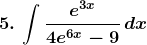Solution: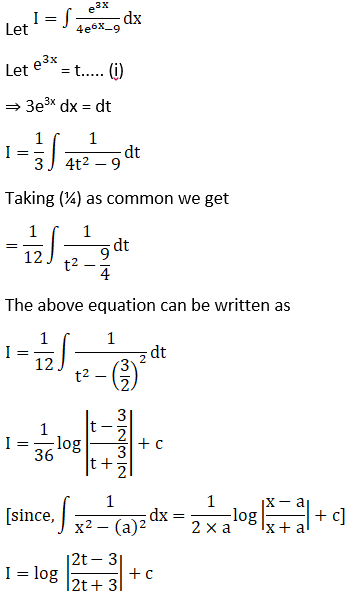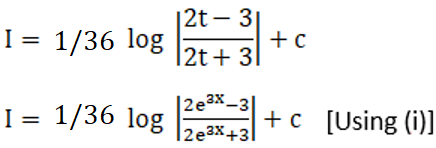### Access other exercises of RD Sharma Solutions For Class 12 Chapter 19 – Indefinite Integrals

Exercise 19.1 Solutions

Exercise 19.2 Solutions

Exercise 19.3 Solutions

Exercise 19.4 Solutions

Exercise 19.5 Solutions

Exercise 19.6 Solutions

Exercise 19.7 Solutions

Exercise 19.8 Solutions

Exercise 19.9 Solutions

Exercise 19.10 Solutions

Exercise 19.11 Solutions

Exercise 19.12 Solutions

Exercise 19.13 Solutions

Exercise 19.14 Solutions

Exercise 19.15 Solutions

Exercise 19.17 Solutions

Exercise 19.18 Solutions

Exercise 19.19 Solutions

Exercise 19.20 Solutions

Exercise 19.21 Solutions

Exercise 19.22 Solutions

Exercise 19.23 Solutions

Exercise 19.24 Solutions

Exercise 19.25 Solutions

Exercise 19.26 Solutions

Exercise 19.27 Solutions

Exercise 19.28 Solutions

Exercise 19.29 Solutions

Exercise 19.30 Solutions

Exercise 19.31 Solutions

Exercise 19.32 Solutions